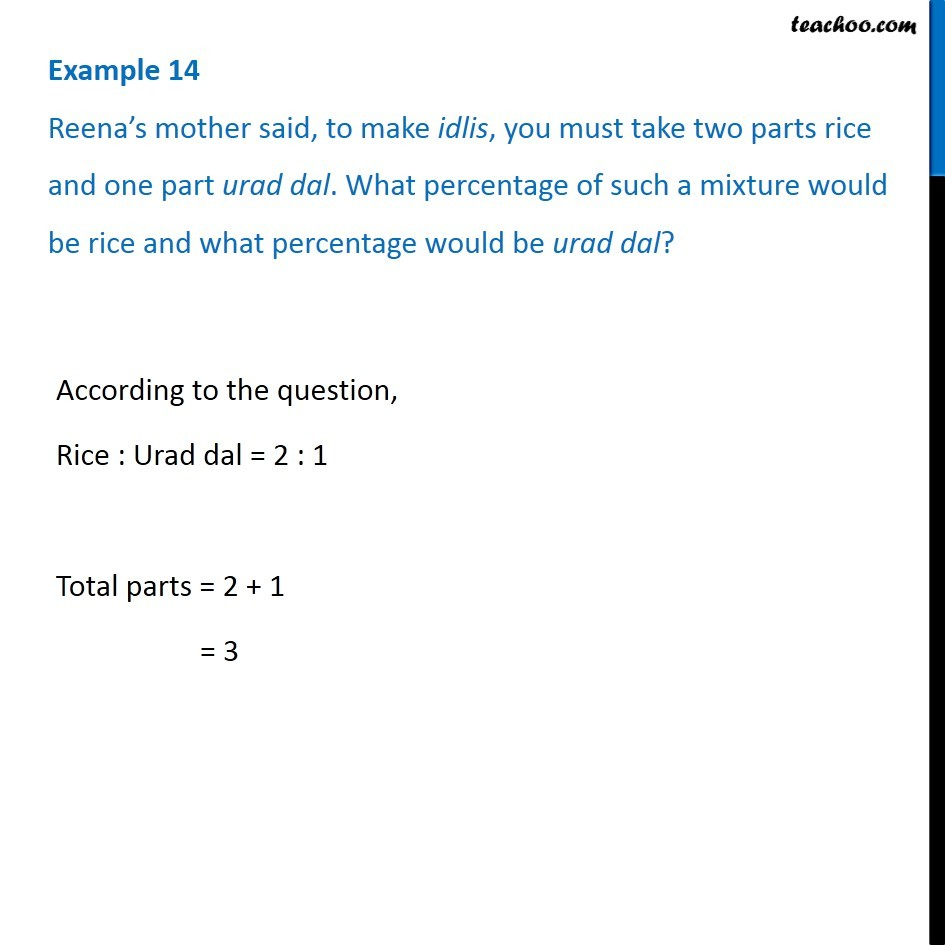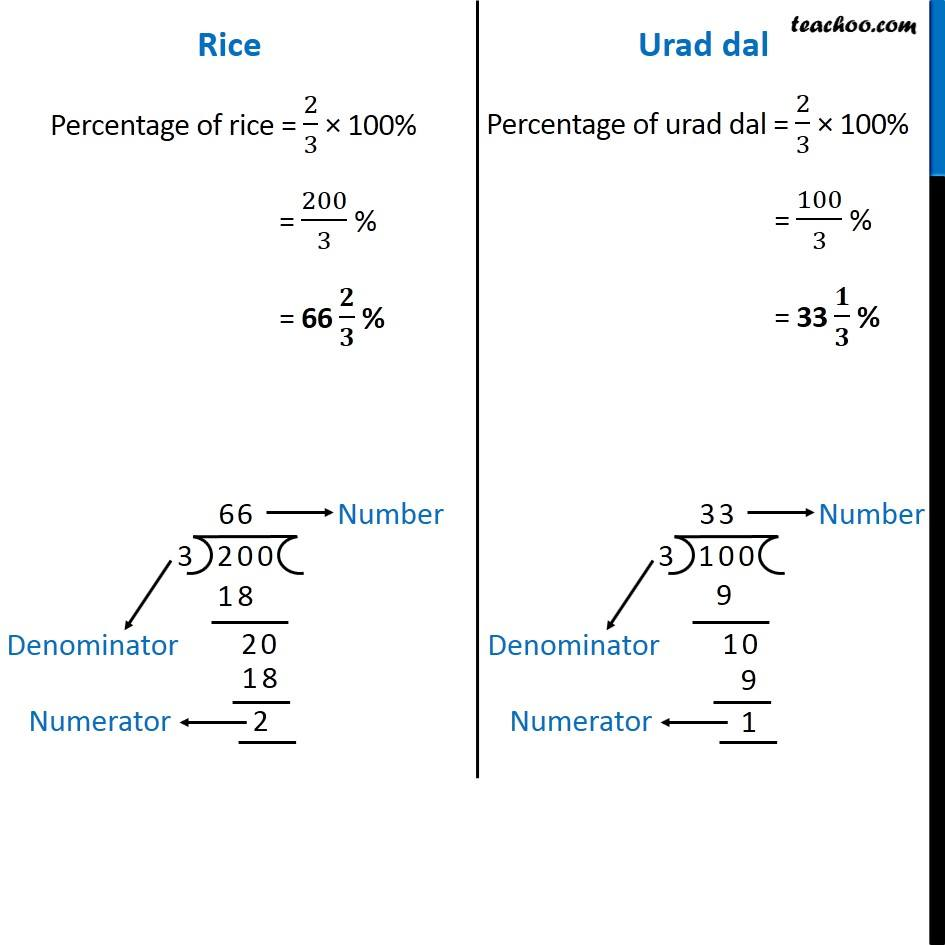Examples

Chapter 8 Class 7 Comparing Quantities
Serial order wiseGet live Maths 1-on-1 Classs - Class 6 to 12

### Transcript

Example 14 Reena’s mother said, to make idlis, you must take two parts rice and one part urad dal. What percentage of such a mixture would be rice and what percentage would be urad dal? According to the question, Rice : Urad dal = 2 : 1 Total parts = 2 + 1 = 3 Rice Percentage of rice = 2/3 × 100% = 200/3 % = 66 𝟐/𝟑 % Urad dal Percentage of urad dal = 2/3 × 100% = 100/3 % = 33 𝟏/𝟑 %